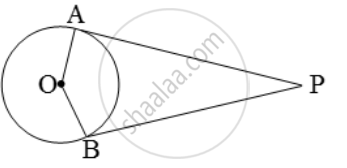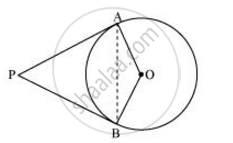# Prove that the Angle Between the Two Tangents Drawn from an External Point to a Circle is Supplementary to the Angle Subtended by the Line Segments Joining the Pointsof Contact to the Centre. - Mathematics

Prove that the angle between the two tangents drawn from an external point to a circle is supplementary to the angle subtended by the line segments joining the pointsof contact to the centre.

#### Solution 1

Given: PA and PB are the tangent drawn from a point P to a circle with centre O. Also, the line segments OA and OB are drawn.To Prove: ∠APB + ∠AOB = 180°

Proof: We know that the tangent to a circle is perpendicular to the radius through the point of contact.

∴ PA ⊥OA ⇒ ∠OAP = 90°, and

PB ⊥ OB ⇒ ∠OBP = 90°.

∴ ∠OAP + ∠OBP = 90°.

Hence, ∠APB + ∠AOB = 180°

[∵ sum of the all the angles of a quadrilateral is 360°]

#### Solution 2Let us consider a circle centered at point O. Let P be an external point from which two tangents PA and PB are drawn to the circle which are touching the circle at point A and B respectively and AB is the line segment, joining point of contacts A and B together such that it subtends ∠AOB at center O of the circle.

It can be observed that

Therefore, ∠OAP = 90°

Similarly, OB (radius) ⊥ PB (tangent)

∠OBP = 90°

Sum of all interior angles = 360º

∠OAP +∠APB+∠PBO +∠BOA = 360º

90º + ∠APB + 90º + ∠BOA = 360º

∠APB + ∠BOA = 180º

Hence, it can be observed that the angle between the two tangents drawn from an external point to a circle is supplementary to the angle subtended by the line-segment joining the points of contact at the centre.

Concept: Number of Tangents from a Point on a Circle
Is there an error in this question or solution?
Chapter 10: Circles - Exercise 10.2 [Page 214]

#### APPEARS IN

NCERT Class 10 Maths
Chapter 10 Circles
Exercise 10.2 | Q 10 | Page 214

Share